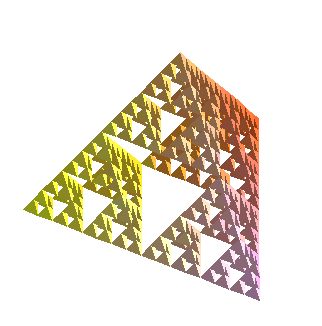## Making a fractal in R3, a Sierpinski pyramid

First, we define some useful routines. Tetra takes a list of the verticies of a tetrahedron, and returns a list of its faces (4 triangles).

```Tetra := proc(verts)
local a,b,c,d;
a := verts;
b := verts;
c := verts;
d := verts;
RETURN( [[a,b,c], [a,b,d], [a,c,d], [b,c,d]]);
end:
```
Midpoint returns the midpoint of the segment joining two points.

```Midpoint := proc(a,b)
RETURN( [(a+b)/2, (a+b)/2, (a+b)/2]);
end:
```
Now for the guts.
Refine takes a tetrahedron (a list of 4 points) and returns the 4 smaller tetrahedra defined by those points and the midpoints of each edge.

```Refine := proc (tetra)
local a,b,c,d;
a:=tetra;
b:=tetra;
c:=tetra;
d:=tetra;
RETURN( [a, Midpoint(a,b), Midpoint(a,c), Midpoint(a,d)],
[b, Midpoint(a,b), Midpoint(b,c), Midpoint(b,d)],
[c, Midpoint(c,b), Midpoint(a,c), Midpoint(c,d)],
[d, Midpoint(d,b), Midpoint(d,c), Midpoint(a,d)]);
end:
```
RefineAll applies Refine to each element of a list of tetrahedra. Note that it uses map. we could have written it with seq, but this is shorter and has the same effect.

```RefineAll := proc(tlist)
RETURN(map(Refine,tlist));
end:
```
Recurse is a general purpose routine that computes the n-fold composition of f applied to the second argument. that is, f(f(f(f(...f(input)...))))

```Recurse := proc(f, input, n)
if ( n <= 0) then
RETURN(input);
else
RETURN(Recurse(f, f(input), n-1));
fi;
end:
```

Now we can put it all together. FirstT is our initial tetrahedron, equilateral of side length 2. I know the height looks funny, but thats it. Really.

```FirstT := [[-1,-1,0],[0,sqrt(3)-1,0],[1,-1,0],[0,sqrt(3)/3-1,2*sqrt(15)/5]]:
```
Here we go. Here's the 3rd stage. (Notice that we have to map Tetra onto each element of the list that comes out of the recursion, so that we will have triangles instead of the verticies of tetrahedra.

```with(plots):
```

```setoptions3d(axes=none, scaling=constrained,shading=XY,style=patch,orientation=[60,48]);
```

```polygonplot3d(map(Tetra,[Refine(FirstT)]),title=`Sierpinski Pyramid, level 1`);
```Things get a little difficult to see when you start refining, although if you make the picture in Maple and can spin it, it helps a lot. Below is level 4, and if you click on the image, you get level 5.

```polygonplot3d(map(Tetra,Recurse(RefineAll,[FirstT],4)),title=`Sierpinski Pyramid, level 4`);
```What would you suspect the dimension of the limiting object is? (remember we started with solid pyramids.) If you do the calculation, you find it is log 4/log2, that is, its 2! so we have a fractal with an integer dimension (its just the "wrong" one, from a topological perspective).